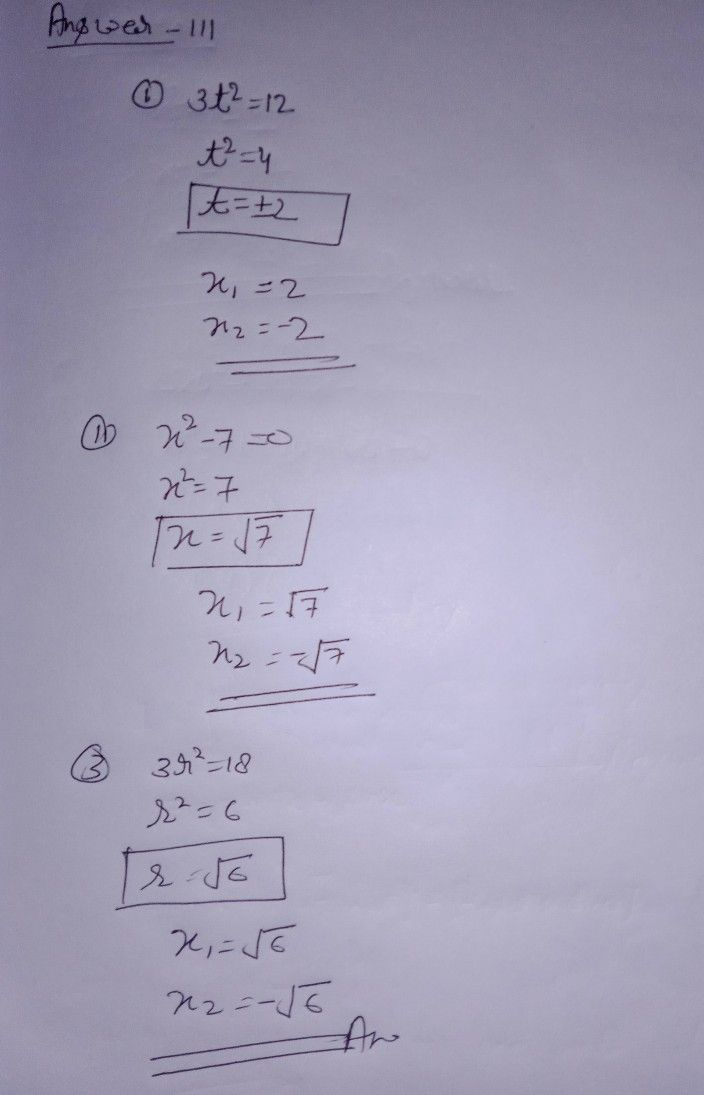Symbol
Problem$111$ Solve each of the following quadratic equations by extracting the square roots. 1. $3t^{2}=12$ X1 = $x_{2}=$ 2. $x^{2}-7=0$ X1 = $x_{2}=$ 3. $3r^{2}=18$ X1 = $x_{2}=$ IV. Solve the following quadratic equations by factoring. 1. $x^{2}+7x=0$ $x_{1}=$ X2 = 2. $t^{2}+8t+16=0$ X1 = $x_{2}=$ = 3. $x^{2}-10x+25=0$ x1 = $x_{2}=$ V. Solve the following quadratic equations by completing the square. 1. $x^{2}-2x=3$ X1 = $x_{2}=$ 2. $s^{2}+4s-21=0$ X1 = X2 D *VI. Solve by using the quadratic formula. 1. $2x^{2}+3x=27$ X1 = $x_{2}=$
7th-9th grade
Algebra
Search count: 117
Question content
may I need your help thankyou
SolutionQanda teacher - henrysee